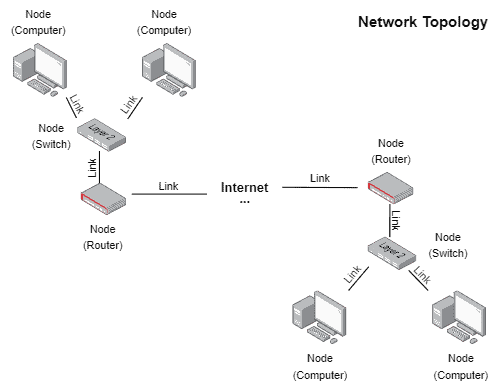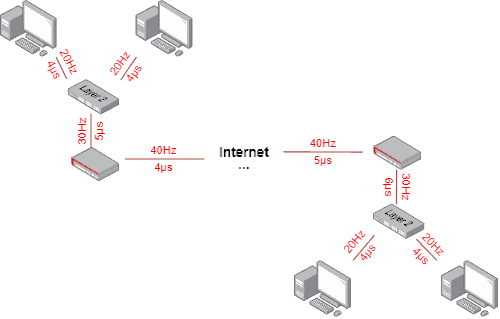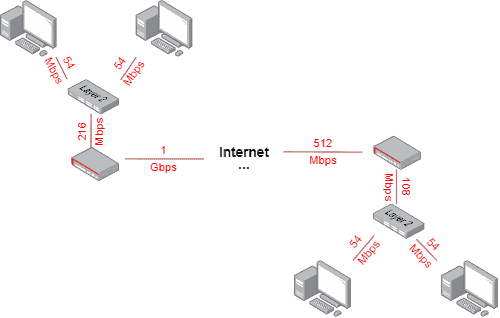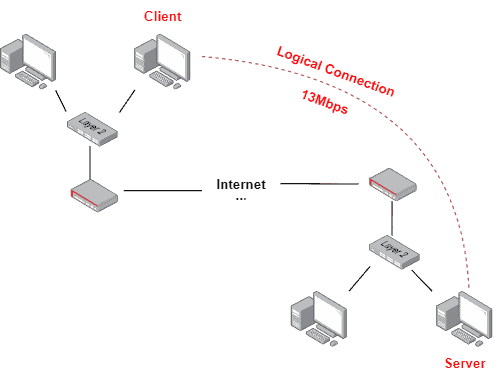## 1. Introduction

Currently, we need to connect to networks for several reasons. As networking becomes more popular daily, many services migrate to networked environments, thus being provided through the Internet.

In such a way, improving the network’s capacity and monitoring the quality of service provided are crucial activities in the modern world. In particular, there are multiple metrics we can consider to understand the capabilities and quality of networks. Among these metrics, we can highlight the link bandwidth, data rate, and throughput.

So, this tutorial explores the previously cited network-related metrics. First, we’ll briefly review, at a high level, what composes a network topology. Thus, we’ll understand the metrics of link bandwidth, data rate, and throughput. Finally, we’ll compare these metrics in a systematic summary.

## 2. Network Topologies

Simply and abstractly, we can see networks as graphs on which nodes represent computers (from user terminals to network functions), and the edges are links employed to steer traffic from one point to another. We call this graph of network topology.

Each node and edge in the graph can represent a particular set of data, defined according to their technical characteristics (static attributes) and the current state of the network (variable attributes).

There are other characteristics and metrics, in turn, defined taking into account several metrics and measurements taken into an entire path of the network topology, for example, when a client communicates with a server (in a client-server architecture). These metrics provide information about the quality of the end-to-end communication itself.

The following figure depicts a network topology example:Thus, with this background information in mind, we can investigate the metrics of link bandwidth, data rate, and throughput in the following sections.

## 3. Bandwidth

Bandwidth consists of a characteristic related to the links of network topology. This characteristic indicates the frequency width supported by a particular link in a network. So, we employ the Hertz (Hz) unit to express bandwidth.

In this way, bandwidth directly influences the data rate and throughput in a networked system since it defines physical limits that can not be transposed.

However, we can not see the relation between bandwidth and data rate or throughput by only analyzing the supported frequency of a specific link. It depends on logical decisions about how to use such frequency width too.

Among these logical decisions, we can cite the employed signal modulation strategy, the number of subchannels available, and the used cycle period. These characteristics with the total bandwidth determine the symbol rate of a system. The symbol rate, in turn, is the time the system needs to transmit a single symbol.

So, it is possible to have links with the same bandwidth but different symbol rates in a network.

The figure next depicts (in red) the bandwidth and symbol rate characteristics in network topology:It is relevant to highlight that, from the OSI model perspective, when we talk about bandwidth, we are talking about the physical layer (layer one).

## 4. Data Rate

In summary, we can say that the data rate refers to the maximum capacity of a link channel to transmit data in a period. Usually, we use bits as data and seconds to indicate the period. Thus, the traditional metric for measuring the data rate of a system is bits per second (bps).

The data rate is a characteristic defined between the data link and the physical layer, considering the OSI model. Thus, it works with raw frames (without including the overload of any forward error correction overload, for example).

Similarly, from a network topology perspective, it is a character defined in the connection of a network node and links since it depends on the bandwidth of the links but also on many logical decisions and strategies adopted to transmit data.

Calculating the data rate will depend on the employed modulation on the networked system, the number of data subchannels, the bit relation between raw data and coded data (regarding the adopted forward error correction method), and the system rate.

Let’s consider single-channel links. So, the following figure exemplifies the data rate characteristic in network topology:Again, varying a single of the previously stated characteristics modify the data rate. Thus, we can have links with the same bandwidth presenting the same bit rate. However, the heterogeneous decision on the employed forward error correction method may make the data rate between nodes connected to such links differ.

## 5. Throughput

Throughput is a generic term employed in multiple cases in computing and networking. In our scenario, we can see throughput as the real data we receive/send in a certain period.

Note that when a system operator triggers a message sending, he indicates the real data (end-to-end significative data). Then, the real data is fragmented into segments, packets, and frames to be sent through the network.

Each protocol used to enable communications between networked entities includes extra data to the real data, which allows, for example, the execution of routing and addresses demultiplexing, among other tasks.

Thus, the capacity to transmit real data (the throughput) is naturally smaller than the data rate since we use part of the data rate to send this extra data (typically presented as a set of headers encapsulating the real data).

We can measure the throughput in the networked nodes in a network topology. We can calculate it as the relation between the real data and the received data in a certain period, thus multiplying it by the expected data rate.

However, transmitting data is a complex process, and we can not guarantee that the data rate always will be achieved. We must consider that other factors besides data overload may affect the throughput too. For example, the necessity of retransmissions of a message and overloaded channels can impact it. So, we should attempt such variable characteristics when calculating and analyzing the throughput.

The following image shows an example of measured throughput in data transmission between two entities belonging to a network topology:## 6. Systematic Summary

Understanding the network capabilities and monitoring performance metrics are crucial for providing efficient and high-quality networked services.

In particular, we have the bandwidth, data rate, and throughput among the several characteristics and metrics we can analyze. Unfortunately, these cause lots of confusion, and sometimes it can be hard to differentiate their meanings.

However, although the metrics of bandwidth, data rate, and throughput are correlated, they also have many differences, as exposed in the following table:## 7. Conclusion

In this tutorial, we studied bandwidth, data rate, and throughput. Initially, we briefly reviewed network topologies to understand better where each studied metric actuates on a network. So, we specifically investigated each metric, finally comparing them in a systematic summary.

We can conclude that bandwidth, data rate, and throughput closely relate but also present different characteristics and aspects of networked systems. In this way, all have particular importance when building and maintaining networks.